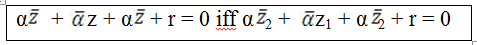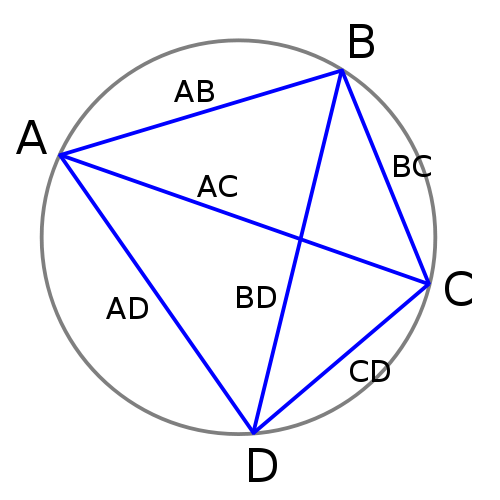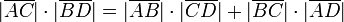×#### Thank you for registering.

One of our academic counsellors will contact you within 1 working day.

Click to Chat

1800-1023-196

+91-120-4616500

CART 0

• 0

MY CART (5)

Use Coupon: CART20 and get 20% off on all online Study Material

ITEM
DETAILS
MRP
DISCOUNT
FINAL PRICE
Total Price: Rs.

There are no items in this cart.
Continue Shopping```Reflection Points for a Straight LineThe concept of reflection assumes different meanings when it is talked about in context of a point or of a straight line.

Point reflection arises when the figure is built around a single point which in that case is termed as the point of reflection. In fact, corresponding to every point in the figure there exists a point which is directly opposite to it on the other side of the center in such a way that the point is indeed the midpoint of the line joining the point and its image.

The concept of reflection points of a straight line or reflection on a number line is important and a bit tricky too. It is entirely different from the point reflection. Two points say P and Q are said to be the reflection points for a given straight line if the given line is the perpendicular bisector of the segment PQ. Note that the two points denoted by the complex numbers z1 and z2 will be the reflection points for the straight linewhere r is real and α is non-zero complex constant.

Inverse points of a circle -

Two points P and Q are said to be inverse w.r.t. a circle with centre 'O' and radius P if

(i) The points O, P, Q are collinear and P, Q are on the same side of O.

(ii) OP.OQ = P2Now, as depicted in the figure above, (OP)(OP’) = r2. Various conclusions follow form this equation like:

If (OP) (OP’) = r2, then (OP’)(OP) = r2.

This means that if point P is inverse of point P’, then the point P’ is the inverse of point P.

In case OP < r, then OP’ > r

If a particular point lies in the interior of the circle of inversion, then its inverse will lie in the exterior.

In case OP > r, then OP’ < r

This case is quite opposite to the previous case. If a particular point lies in the exterior of the circle of inversion, then its inverse will lie in the interior.

In case OP = r, then OP’ = r

Now, if the point lies on the circle of inversion itself then so does its inverse. Hence, the point in such a case may be regarded to be invariant. This means that the point is its own inverse.

Remark: Mathematically, the inverse points may also be defined as:

Two points z1 and z2 will be the inverse points w.r.t. the circleThe concept of inverse points may also be considered in context of an inversion sphere. The inversion sphere may also be regarded as the extension of the geometric inversion form two dimensional plane to three dimensional space.

Ptolemy's Theorem

Ptolemy theorem is basically a relation between the four sides and the two diagonals of a cyclic quadrilateral. A cyclic quadrilateral is the one whose vertices exist on a common circle.A cyclic quadrilateral with vertices as A, B, C and DHere, the bars denote the lengths of the line segments.

Let us discuss the proof of the theorem in detail:

Proof: Consider the cyclic quadrilateral ABCD and extend CD to P in such a way that ∠BAD = ∠CAP.

The quadrilateral ABCD is cyclic, so m ∠ABC + m ∠ADC = 180°. But, we know that ∠ADP forms a linear pair with ∠ADC. Hence, this implies that ∠ADP = ∠ABC.

This gives that ? ABC~ ? ADP (by AA similarity).

Now, AB/AD = BC/DP which means that DP = (AD) (BC)/ (AB).

Since the two angles subtend the same arc, so we have ∠ABD = ∠ACD. Moreover, ∠BAC + ∠CAD = ∠DAP + ∠CAD.

By cancelling the common angle on both sides we get,

Hence, ? BAD ~ ? CAP.

This gives AB/AC = BD/CP. Hence, CP = (AC) (BD)/ (AB).

But, CP = CD + DP. Making the required substitutions, we get

(AC) (BD)/ (AB) = CD + (AD)(BC)/ (AB).

Multiplying by AB on both sides gives,

It may also be stated in another way. It says that the product of the lengths of the diagonals of a converse quadrilateral inscribed in a circle is equal to the sum of the products of lengths of the two pairs of its opposite sides, i.e.,

|z1-z3| |z2-z4| = |z1-z2| |z3-z4|+|z1-z4| |z2-z3|

Ptolemy’s Inequality: Another result that follows from the Ptolemy’s theorem is the Ptolemy’s inequality. If A, B, C and D are any four points which are not necessarily concyclic then,askIITians offers comprehensive study material which contains all the important topics of IIT JEE Mathematics. Questions like how to find out reflection of point with respect to line or inverse points of a circle or reflections of complex numbers have been aptly answered in the material. It is important to have thorough knowledge of this topic in order to remain competitive in the JEE.

Related Resources

Look into the Past Year Papers with Solutions to get a hint of the kinds of questions asked in the exam.

You can get the knowledge of Useful Books of Mathematics here.

To read more, Buy study materials of Complex Numbers comprising study notes, revision notes, video lectures, previous year solved questions etc. Also browse for more study materials on Mathematics here.
```### Course Features

• 731 Video Lectures
• Revision Notes
• Previous Year Papers
• Mind Map
• Study Planner
• NCERT Solutions
• Discussion Forum
• Test paper with Video Solution